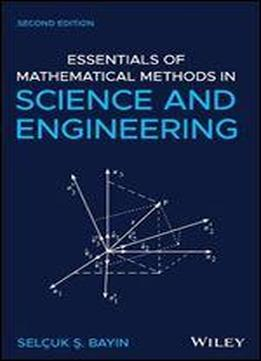# Essentials Of Mathematical Methods In Science And Engineering by Selcuk S. Bayin / 2020 / English / PDF

A comprehensive introduction to the multidisciplinary applications of mathematical methods, revised and updated The second edition of Essentials of Mathematical Methods in Science and Engineering offers an introduction to the key mathematical concepts of advanced calculus, differential equations, complex analysis, and introductory mathematical physics for students in engineering and physics research. The books approachable style is designed in a modular format with each chapter covering a subject thoroughly and thus can be read independently. This updated second edition includes two new and extensive chapters that cover practical linear algebra and applications of linear algebra as well as a computer file that includes Matlab codes. To enhance understanding of the material presented, the text contains a collection of exercises at the end of each chapter. The author offers a coherent treatment of the topics with a style that makes the essential mathematical skills easily accessible to a multidisciplinary audience. This important text: Includes derivations with sufficient detail so that the reader can follow them without searching for results in other parts of the book Puts the emphasis on the analytic techniques Contains two new chapters that explore linear algebra and its applications Includes Matlab codes that the readers can use to practice with the methods introduced in the book Written for students in science and engineering, this new edition of Essentials of Mathematical Methods in Science and Engineering maintains all the successful features of the first edition and includes new information.

views: 366